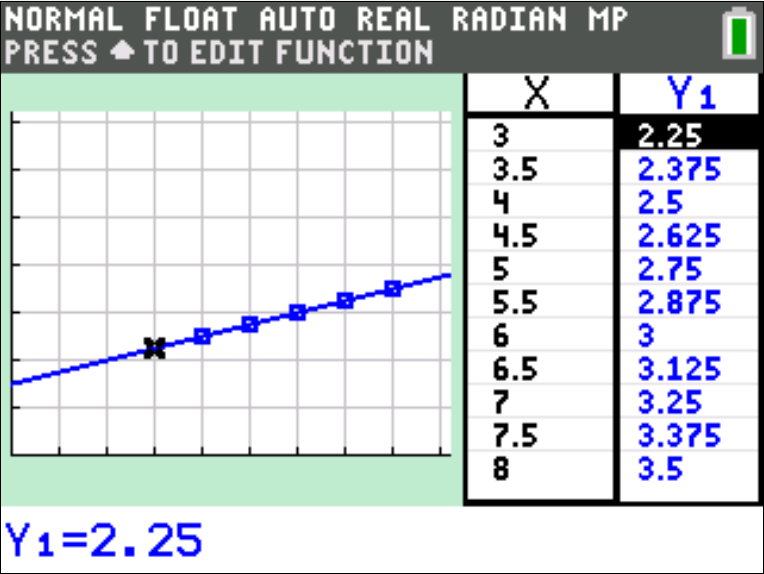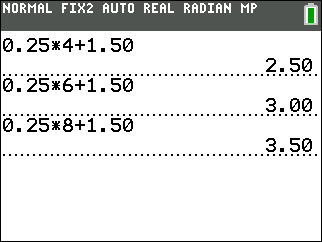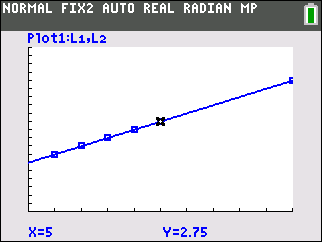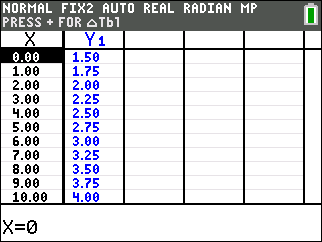# Activities

••• ##### Subject Area

• Math: Middle Grades Math: Algebraic Thinking

• ##### Author6-8

45 Minutes

• ##### Device
• TI-83 Plus Family
• TI-84 Plus
• TI-84 Plus Silver Edition
•TI-84 Plus C Silver Edition
•TI-84 Plus CE

TI Connect™

## The Variables of Renting#### Activity Overview

In this activity, students will identify what a variable is, construct a table of values, graph the ordered pairs from the table, and use the TI-84 Plus C Silver Edition to graph the equation.

#### Key Steps

•Students will identify what a variable is, construct a table of values, graph the ordered pairs from the table, and use the TI-84 Plus C Silver Edition to graph the function. First, students look at the video rental rates using number sentences.

•Then, students graph the scatter plot of ordered pairs and look at the line that passes through all the points.

•Finally, students analyze the table of function values generated from function representing the video rental scenario.